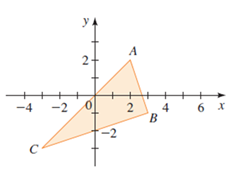# To verify: The triangle A B C is a right triangle using converse of Pythagoras theorem.### Precalculus: Mathematics for Calcu...

6th Edition
Stewart + 5 others
Publisher: Cengage Learning
ISBN: 9780840068071### Precalculus: Mathematics for Calcu...

6th Edition
Stewart + 5 others
Publisher: Cengage Learning
ISBN: 9780840068071

#### Solutions

Chapter 1.8, Problem 39E

(a)

To determine

## To verify: The triangleABC is a right triangle using converse of Pythagoras theorem.

Expert Solution

### Explanation of Solution

Given information:

The figure,Formula used:

Converse of Pythagoras theorem states that if the sum of squares of two sides of a triangle is equal to the square of longest side of the triangle, then the triangle is a right triangle.

Distance formula between two points X(a1,b1) and Y(a2,b2) is calculated as,

d(XY)=(a2a1)2+(b2b1)2

Proof:

Consider the given figure,In the above figure, the coordinates of the vertices of the triangle ABC are A(2,2),B(3,1) and C(3,3).

Recall that the distance formula between two points X(a1,b1)and Y(a2,b2)is calculated as,

d(XY)=(a2a1)2+(b2b1)2

So, length of AB will be calculated as.

d(A,B)=(32)2+(12)2=(1)2+(3)2=1+9=10

Now, the length of BC will be calculated as,

d(B,C)=(33)2+(3+1)2=(6)2+(2)2=36+4=40

Length of AC will be calculated s,

d(A,C)=(32)2+(32)2=(5)2+(5)2=25+25=50

Now, calculate the sum of squares of AB and BC as,

(d(A,B))2+(d(B,C))2=(d(A,C))2(10)2+(40)2=(50)210+40=5050=50

Recall the converse of Pythagoras theorem if the sum of squares of two sides of a triangle is equal to the square of longest side of the triangle, then the triangle is a right triangle.

Here, square of longest side i.e. AC is equal to the sum of squares of other two sides, i.e. AB and BC, so, the given triangle ABC is a right triangle.

Thus, using converse of Pythagoras theorem it is proved that the triangle ABC is a right triangle.

(b)

To determine

### To calculate: The area of triangle ABC.

Expert Solution

The area of triangle ABC is 10 sq. units.

### Explanation of Solution

Given information:

The figure,Formula used:

Area of a triangle is half into the product of its base and height. So, if b is the base and h is the height of the triangle, then area of triangle is expressed as,

Area=12bh

Calculation:

Consider the given figure,In (a) part, lengths of sides of triangle are calculated as,

Length of AB =10 units.

Length of BC =40 units.

Length of AC =50 units.

Since, AC is longest, so, it is hypotenuse and AB is shortest, so, AB is the perpendicular (height) and BC is base of the triangle.

Recall area of a triangle is half into the product of its base and height. So, if b is the base and h is the height of the triangle, then area of triangle is expressed as,

Area=12bh

Apply it,

Area=12bh=12(40)(10)=12(400)

Simplify it further as,

Area=12(400)=12(20)=10 sq. units

Thus, area of the triangle ABC is 10 sq. units.

### Have a homework question?

Subscribe to bartleby learn! Ask subject matter experts 30 homework questions each month. Plus, you’ll have access to millions of step-by-step textbook answers!